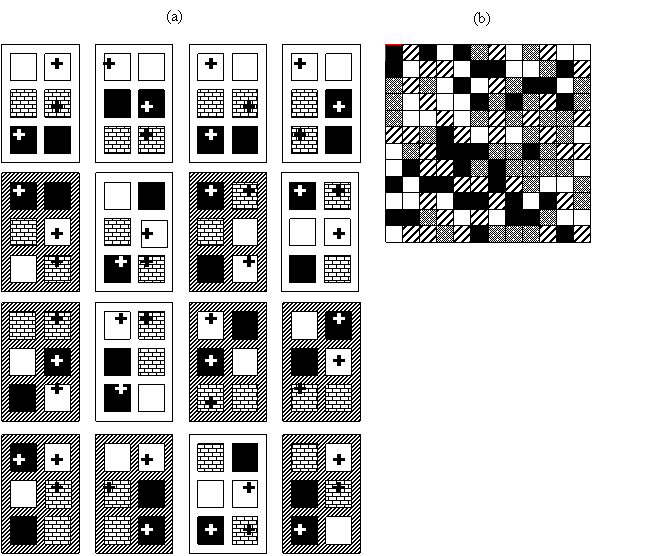### Katherine L. Gross, Gary G. Mittelbach, and Heather L. Reynolds. 2005. Grassland invasibility and diversity: responses to nutrients, seed input, and disturbance. Ecology 86:476–486.

Appendix A. A diagram of experimental design (Fig. A1a, b) and a table (Table A1) specifying the error terms used to test main effects and interactions in our modified split-plot design.FIG. A1. (a) The experimental design consisted of eight undisturbed (open) and eight disturbed (cross-hatched) blocks in which vegetative cover was reduced in early April 2000. Each block included six 3 × 3 m seed addition × fertilization plots (‘+’s’ indicate seed added; open, patterned, and dark fill indicate control, patchy, and uniform fertilization, respectively). Plots within blocks were separated by 1-m walkways; blocks were separated by 5-m unmanipulated vegetation. (b) Example of grid cell level (25 × 25 cm) fertilization treatments in a patchy fertilization plot. Each grid cell was randomly assigned to one of four fertilizer levels: None = open fill, Low = lined fill, Medium = dotted fill and High = dark fill.

TABLE A1. The ANOVA Model used to test for main effects and interactions: Y = constant + cover reduction (R) + seed addition (S) + fertilizer (F) + (R × S) + (R × F) + (F × S) + (R × S × F) + Block (R) + (S × Block (R)) + (F × Block (R)).

 Effects df Error term Between subjects 1 Reduced cover (R) 1 Block (R) Seed additiond (S) 2 S × Block (R) Fertilizer (F) 1 F × Block (R) R × S 2 S × Block (R) R × F F × Block (R) Within subjects S × F 2 MS error (ANOVA table) R × S × F 2 MS error (ANOVA table)

[Back to E086-024]